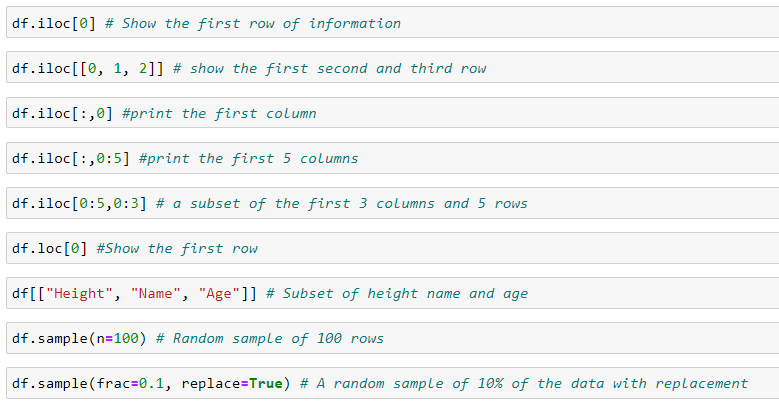# Part 6: Data Cleaning

## Step 1: Understanding the Data

Before cleaning data, there are a couple of things we would like to know: for example, the dimensions of a dataset, the data type of each variable, perhaps a peek at the first few rows and last few rows of the data (to see what it looks like and confirm it matches our expectations), the name of each variable, etc.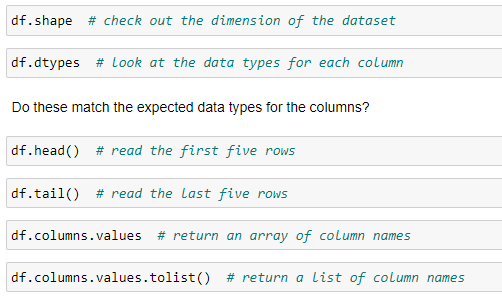## Step 2: Check Missing Values

Next, we would like to check if there are any missing values. To check this, we can use the function dataframe.isnull() in pandas. It will return `True` for missing components and `False` for non-missing cells. However, when the dimension of a dataset is large, it could be difficult to figure out the existence of missing values. In general, we may just want to know if there are any missing values at all before we try to find where they are. The function dataframe.isnull().values.any() returns True when there is at least one missing value occurring in the data. The function dataframe.isnull().sum().sum() returns the number of missing values in the dataset.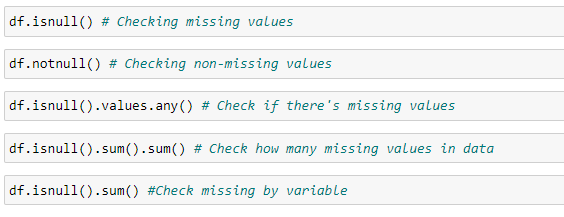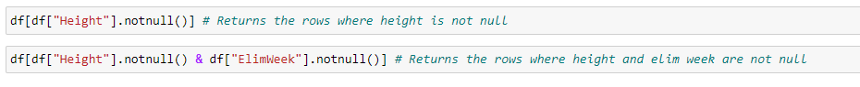## Step 3: Get Information about Missing Values

We could subset the data based on the missing values and create a new data frame to hold all the rows.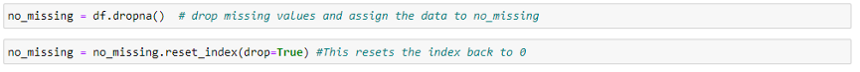You can also set a threshold of missing values. In the below example it drops rows that contain less than 50 non-missing values.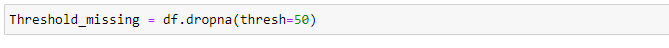If we use dataframe.dropna(thresh=25) to drop rows that contain less than 25 non-missing values, we don't change the original data. We can assign the output to a new variable or save the changes to the original data right away by using dataframe.dropna(thresh=25, inplace=True). For our example, it would be df.dropna(thresh=25, inplace=True).

## Step 4: Fill In Missing Values

For quantitative variables, we may replace missing values with the sample mean, mode, median, or other numbers. For categorical variables, we can create a new category for missing values by replacing missing values with a string.

Replace missing values with 0.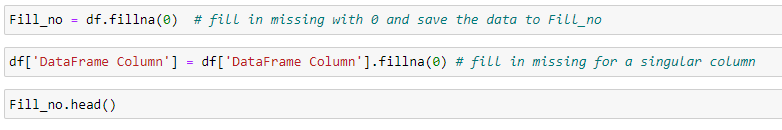Replace all missing with string missing.## Step 5: Dropping Data

We may want to drop duplicate rows if any and save the changes to the original data.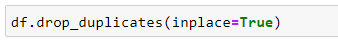We also may want to drop some observations or some columns.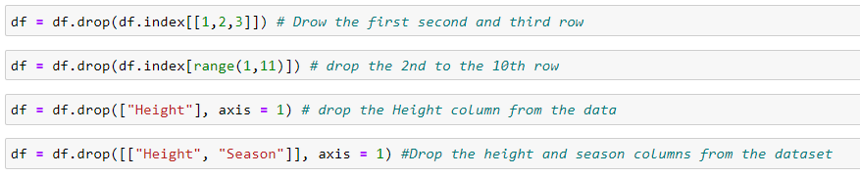## Step 6: Subsetting

iloc stands for integer location. It helps subset data by using integers. It’s counterpart loc uses strings to find data within your data set.